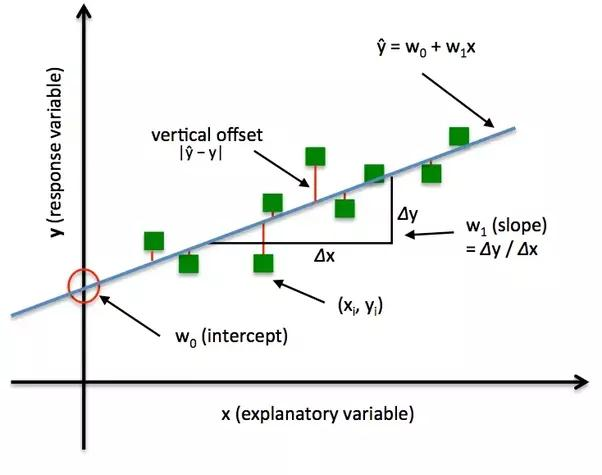/  Deep Learning Interview questions and answers   /  Explain different Loss functions that we generally use for Neural Networks?## Explain different Loss functions that we generally use for Neural Networks?

Ans: There are no specific loss functions used for Neural Networks. In general, we use Classification Loss Functions in Neural Networks.As the name suggests, mean square error is measured as the average of squared difference between predictions and actual observations. It’s only concerned with the average magnitude of error irrespective of their direction. However, due to squaring, predictions which are far away from actual values are penalized heavily in comparison to less deviated predictions. The higher this value, the worse the model is. It is never negative, since we’re squaring the individual prediction-wise errors before summing them, but would be zero for a perfect model.

Note that if we want to have a constant prediction the best one will be the mean value of the target values. It can be found by setting the derivative of our total error with respect to that constant to zero, and find it from this equation.

(b)Mean Absolute Error/L1 LossMean absolute error, on the other hand, is measured as the average of sum of absolute differences between predictions and actual observations. Like MSE, this as well measures the magnitude of error without considering their direction. Unlike MSE, MAE needs more complicated tools such as linear programming to compute the gradients. MAE is more robust to outliers since it does not make use of square.

Another important thing about MAE is its gradients with respect to the predictions. The gradient is a step function and it takes -1 when Y_hat is smaller than the target and +1 when it is larger.

Now, the gradient is not defined when the prediction is perfect, because when Y_hat is equal to Y, we cannot evaluate gradient. It is not defined.

(c) Mean Bias Error

This is much less common in machine learning domain as compared to its counterpart. This is same as MSE with the only difference that we don’t take absolute values. Clearly there’s a need for caution as positive and negative errors could cancel each other out. Although less accurate in practice, it could determine if the model has positive bias or negative bias.(d) Root Mean Squared Error (RMSE)

RMSE is just the square root of MSE. The square root is introduced to make scale of the errors to be the same as the scale of targets.Now, it is very important to understand in what sense RMSE is similar to MSE, and what is the difference.

First, they are similar in terms of their minimizers, every minimizer of MSE is also a minimizer for RMSE and vice versa since the square root is a non-decreasing function. For example, if we have two sets of predictions, A and B, and say MSE of A is greater than MSE of B, then we can be sure that RMSE of A is greater RMSE of B. And it also works in the opposite direction.

(e) Binary Cross Entropy

Binary cross entropy is a loss function used on problems involving binary decisions. For instance, in multi-label problems, where an example can belong to multiple classes at the same time, the model tries to decide for each class whether the example belongs to that class or not. In neural networks we should use sigmoid function as an activation function at the output layer.(f) Negative Log Likelihood

The negative log-likelihood loss function is often used in combination with a SoftMax activation function to define how well your neural network classifies data.

The negative log-likelihood function is defined as loss=-log(y) and produces a high value when the values of the output layer are evenly distributed and low. In other words, there’s a high loss when the classification is unclear. It also produces relative high values when the classification is wrong. When the value of the output layer matches that of the expected value, the negative log-likelihood function produces a very low value.